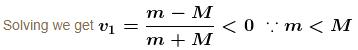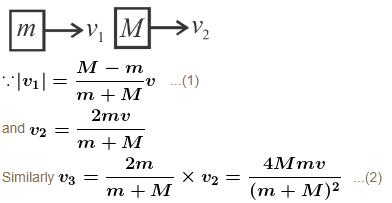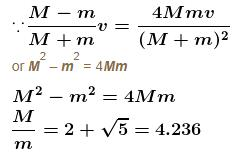Courses

# Centre Of Mass NAT Level - 2

## 10 Questions MCQ Test Basic Physics for IIT JAM | Centre Of Mass NAT Level - 2

Description
This mock test of Centre Of Mass NAT Level - 2 for Physics helps you for every Physics entrance exam. This contains 10 Multiple Choice Questions for Physics Centre Of Mass NAT Level - 2 (mcq) to study with solutions a complete question bank. The solved questions answers in this Centre Of Mass NAT Level - 2 quiz give you a good mix of easy questions and tough questions. Physics students definitely take this Centre Of Mass NAT Level - 2 exercise for a better result in the exam. You can find other Centre Of Mass NAT Level - 2 extra questions, long questions & short questions for Physics on EduRev as well by searching above.
*Answer can only contain numeric values
QUESTION: 1

### Block A is hanging from a vertical spring and it at rest. Block B strikes the block A with velocity v and sticks to it. The the value of v in m/s for which the spring just attains natural length is :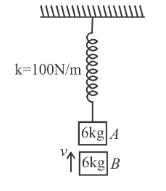Solution:

The initial extension in spring is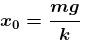Just after collision of B with A the speed of combined mass is  v/2.

For the spring to just attain natural length of combined mass must rise up by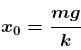(see figure.) and comes to rest.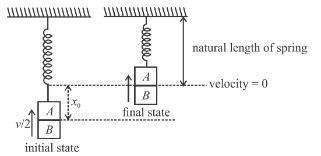Applying conservation of energy between initial and final states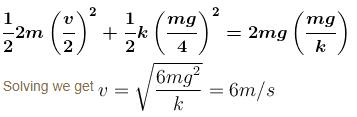Alternate solution by SHM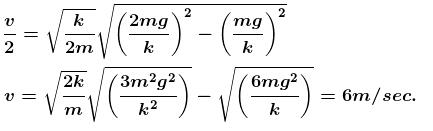*Answer can only contain numeric values
QUESTION: 2

### In the figure shown a small block B of mass m is released from the top of a smooth movable wedge A of the same mass m. The height of wedge A shown in figure is h = 100cm. B ascends another movable smooth wedge C of the same mass. Neglecting friction any where find the maximum height (in cm) attained by block B on wedge C.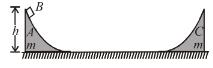Solution: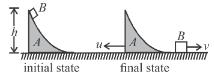Let u and v be speed of wedge A and block B at just after the block B gets off the wedge A. Applying conservation of momentum in horizontal direction, we get.

mu = mv      ...(1)

Applying conservation of energy between initial and final state as shown in figure (1), we get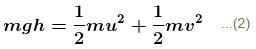solving (1) and (2) we get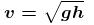...(3)

At the instant block B reaches maximum height h' on the wedge C(figure 2), the speed of block B and wedge C are v'.

Applying conservation of momentum in horizontal direction, we get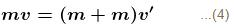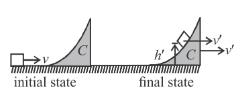Applying conservation of energy between initial and final state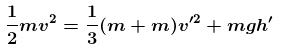Solving equations (3), (4) and (5) we get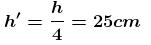*Answer can only contain numeric values
QUESTION: 3

### Each of the block shown in figure has mass 1 kg. The near block moves with a speed of 2 m/s towards the front block kept at rest. The spring attached to the front block is light and has a spring constant 50 N/m. Find the maximum compression of the spring. (in cm) :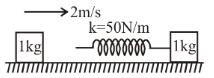Solution:

Maximum compression will take place when the blocks move with equal velocity. As no net external horizontal force acts on the system of the two blocks, the total linear momentum will remain constant. If v is the common speed at maximum compression, we have,

(1 kg) (2m/s) = (1kg)v + (1kg)v

or v = 1m/s

Initial kinetic energy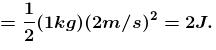Final kinetic energy =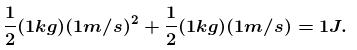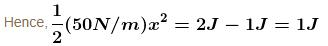or x = 0.2m

*Answer can only contain numeric values
QUESTION: 4

A stationary body explodes into four identical fragments such that three of them fly off mutually perpendicular to each other, each with same kinetic energy., E0. The energy of explosion is given by nE0. Find the value of n.

Solution:

Let the three mutually perpendicular direction be along xy and z-axis respectively.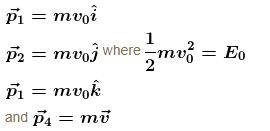By linear momentum conservation,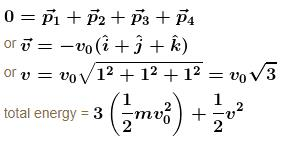= 3E0 + 3E0
= 6E0

*Answer can only contain numeric values
QUESTION: 5

A balloon having mass m is filled with gas and is held in hands of a boy. Then suddenly it get released and gas start coming out of it with a constant rate. The velocities of the ejected gases is also constant 2m/s with respect to the balloon. Find out the velocity of the balloon when the mass of gas is reduced to half. [Effect of atmosphere and gravity is neglected]

Solution:

Neglecting gravity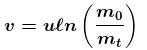u = ejection velocity w.r.t. balloon
m0 = initial mass
m1 = mass at any time t.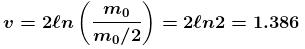*Answer can only contain numeric values
QUESTION: 6

Particle A moves with speed 10 m/s in a frictionless circular fixed horizontal pipe of radius 5 m and strikes with B of double mass that of A. Coefficient of restitution is 1/2 and particle A starts its journey at t = 0. The time at which second collision occurs is given by nπ sec. Find the value of n.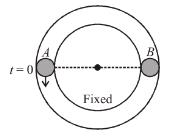Solution:

For first collision v = 10 m/s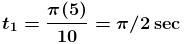velocity of separation = e velocity of approach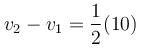v2 – v1 = 5m/s
for second collision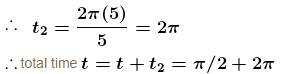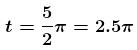*Answer can only contain numeric values
QUESTION: 7

A plate of mass M is moved with constant velocity v against dust particles moving with velocity u in opposite direction as shown. The density of the dust is ρ and plate area is A. Find the force F required to keep the plate moving uniformly is Aρ(u + v)N, then N is :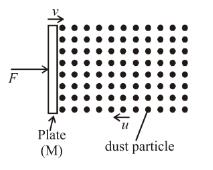Solution:

Force F on plate = force exerted by dust particles
= force on dust particles by the plate
= rate of change of momentum of dust particles
= mass of dust particles striking the plate per unit time × change in velocity of dust particles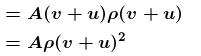*Answer can only contain numeric values
QUESTION: 8

Three ball AB and C (mA = mC = 4 mB) are placed on a smooth horizontal surface. Ball B collides with ball C with an initial velocity v as shown in the figure. Total number of collision between the balls will be : (All collisions are elastic)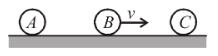Solution:

For 1st collision
Since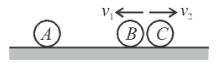e = 1
v = v1 + v2
⇒ v2 = v – v1   ...(1)
By momentum conservation :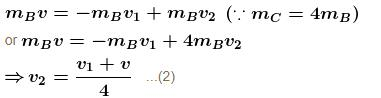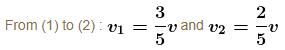For second collision :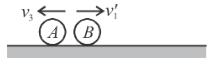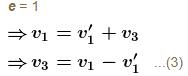By momentum conservation :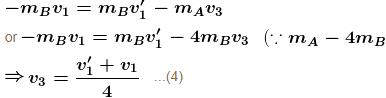From (2) and (4) :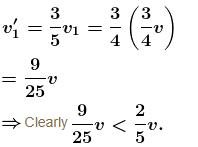Therefore B can not collide with C for the second time.
Hence; total number of collision is 2.

*Answer can only contain numeric values
QUESTION: 9

A particle moving on a smooth horizontal surface strikes a stationary wall. The angle of strikes is equal to the angle of rebound and is equal to 37° and the coefficient of restitution with wall is e = 1/5. Find the friction coefficient between wall and the particle in the form X/10. What is the value of X.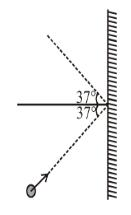Solution:

Since e = 1/5

∴  Final normal component of velocity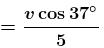As the angle of rebound is equal to the angle before impact.

Therefore, both normal and tangential components of velocities must change by the same factor,
∴ Tangential velocity after impact becomes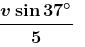Let the time of impact be Δt.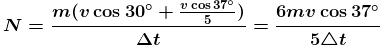where N is the normal force imparted on the ball by the wall.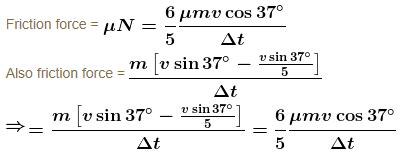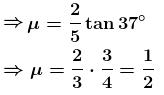*Answer can only contain numeric values
QUESTION: 10

Three blocks are placed on smooth horizontal surface and lie on same horizontal straight line. Block 1 and block 3 have mass m each and block 2 has mass M(M > m). Block 2 and block 3 are initially stationary, while block 1 is initially moving towards block 2 with speed v as shown. Assume that all collisions are headon and perfectly elastic. What value of M/m ensures that block 1 and block 3 have the same final speed?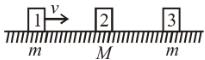Solution:

Let v1v2 and v3  be velocities of block 1, 2 and 3 after suffering collision each.
mv = mv1 + Mv2
and v1 – v2 = –v
v1 – v2 = –v